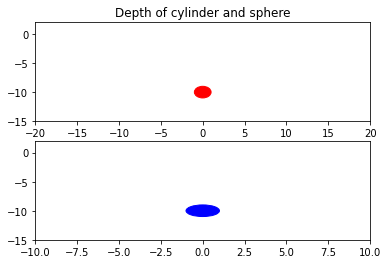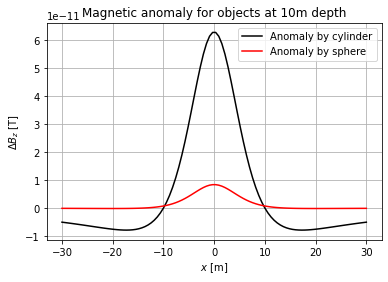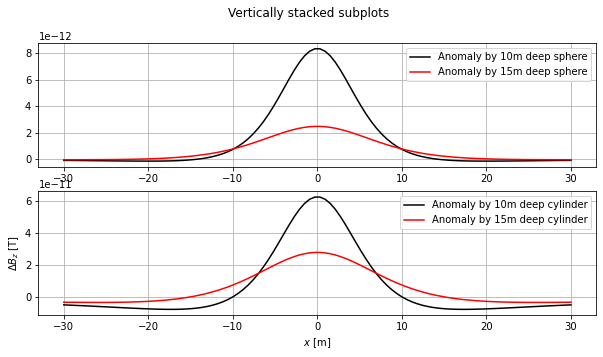# Magnetic surveys#

We are going to examine the magnetic anomaly, $$\Delta B_z$$, caused by a sphere and a horizontal cyclinder at $$10\,m$$ depth. For that, Poissons relation will be used. For this exercise, it is assumed that the magnetic field of the Earth, $$H$$, as well as the magnetic fields from the objects, $$B$$, are parallel. This is being done as follows:

import numpy as np
import matplotlib.pyplot as plt

# Depth of the objects
z_sphere1 = 10.0 # in [m]
z_cylinder1 = 10.0 # in [m]

R_sphere=1.0 # in [m]
R_cylinder=1.0 # in [m]

sphere = plt.Circle((0, -z_sphere1), R_sphere, color='r')
cylinder = plt.Circle((0, -z_cylinder1), R_cylinder, color='blue')

fig, ax = plt.subplots(2)
ax.set_title('Depth of cylinder and sphere')
ax.set_xlim(-20,20)
ax.set_ylim(-15,2)
ax.set_xlim(-10,10)
ax.set_ylim(-15,2)

<matplotlib.patches.Circle at 0x1a96875bf40>Defining the vertical magnetic density gradient is done using Poisson’s relation. For a sphere, this is being done using the equation

$B_{sphere} = \frac{\mu}{3} R_{sphere}^3 dM_z \frac{2 z_{sphere}^2 - x^2}{(z_{sphere}^2 + x^2)^{5/2}}$
$B_{cylinder} = \frac{\mu}{2}R_{cylinder}^2 dM_z \frac{ z_{cylinder}^2 - x^2}{(z_{sphere}^2 + x^2)^2}$
# Magnetic permeability constant
mu=4.0*np.pi*10e-7

# Magnetization contrast [A/m]
dMz = 1.0e-3

# calculating B field of magnetisation
x=np.linspace(-30.0,30.0,100)
dBz1_sphere=(mu/3.0)*R_sphere**3*dMz*(2.0*z_sphere1**2-x**2)/(z_sphere1**2+x**2)**(5.0/2.0)
dBz1_cylinder=(mu/2.0)*R_cylinder**2*dMz*(z_cylinder1**2-x**2)/(z_cylinder1**2+x**2)**2

plt.xlabel(r'$x$ [m]')
plt.ylabel(r'$\Delta B_z$ [T]')
plt.title('Magnetic anomaly for objects at 10m depth')
plt.plot(x,dBz1_cylinder,'k', label = 'Anomaly by cylinder')
plt.plot(x,dBz1_sphere,'r', label = 'Anomaly by sphere')
plt.legend()
plt.grid()
plt.show()Examining the above plot with regards to $$\Delta B_z$$, it can be seen that the magnetic anomaly very much depends on the shape of the object.

Now, we are going to examine what happens if both objects were lying deeper.

z_sphere2 = 15.0 # in [m]
z_cylinder2 = 15.0 # in [m]

x=np.linspace(-30.0,30.0,100)
dBz2_sphere=(mu/3.0)*R_sphere**3*dMz*(2.0*z_sphere2**2-x**2)/(z_sphere2**2+x**2)**(5.0/2.0)
dBz2_cylinder=(mu/2.0)*R_cylinder**2*dMz*(z_cylinder2**2-x**2)/(z_cylinder2**2+x**2)**2

plt.xlabel(r'$x$ [m]')
plt.ylabel(r'$\Delta B_z$ [T]')
plt.title('Magnetic anomaly for objects at 15m depth')
plt.plot(x,dBz2_cylinder,'k', label = 'Anomaly by cylinder')
plt.plot(x,dBz2_sphere,'r', label = 'Anomaly by sphere')
plt.legend()
plt.grid()
plt.show()## To compare
fig, axs = plt.subplots(2, figsize = (10,5))

fig.suptitle('Vertically stacked subplots')
axs.plot(x,dBz1_sphere,'k', label = 'Anomaly by 10m deep sphere')
axs.plot(x,dBz2_sphere,'r', label = 'Anomaly by 15m deep sphere')
plt.xlabel(r'$x$ [m]')
plt.ylabel(r'$\Delta B_z$ [T]')

axs.plot(x,dBz1_cylinder,'k', label = 'Anomaly by 10m deep cylinder')
axs.plot(x,dBz2_cylinder,'r', label = 'Anomaly by 15m deep cylinder')
axs.legend()
axs.legend()

plt.xlabel(r'$x$ [m]')
plt.ylabel(r'$\Delta B_z$ [T]')
axs.grid()
axs.grid()We can observe that the deeper the object, the smaller the magnetic anomaly becomes as well as more spread out.